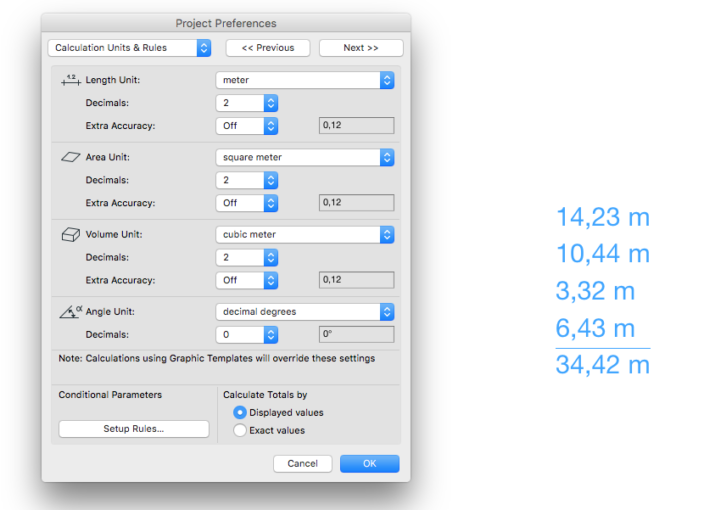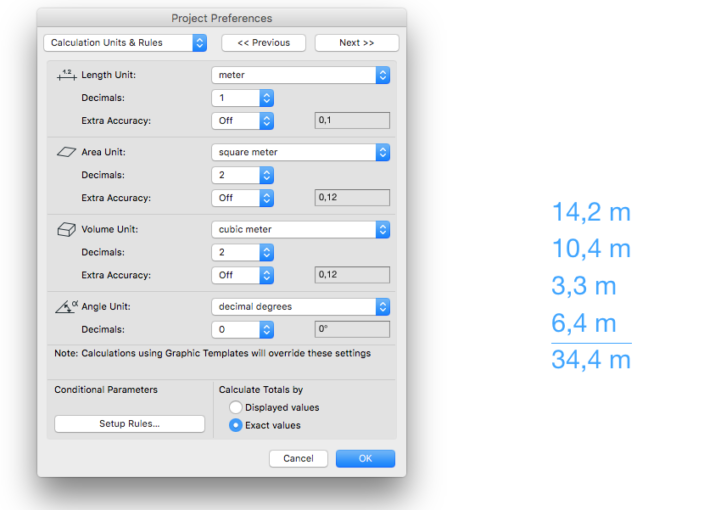## 2 ways of summarizing values

In Architectural plans, the calculated Area, Volume, Length values are usually displayed in a rounded form. However, ARCHICAD stores these values with a high punctuality (12 decimals), therefore in further calculations the result will be punctual, but may differ from the summary of the displayed rounded values.

Certain standards specify that the calculated summaries should be equal to the summary of the displayed values. From ARCHICAD 20 it is possible for the User to decide how to calculate the totals by the Calculate Totals option in Project Preferences/Calculation Units & Rules:The Library Parts can summarize values according to the Project Preferences settings with the “Sum_with_rounding” request.

This request requires that the values are formatted into strings according to one of the Project Preferences settings. For details about this method go to How to display values with units set in Project Preferences post.

In the following example, the values are formatted according to Project Preferences/Calculation Units & Rules/Length Unit settings, therefore the “Calc_length_unit” request is used:

```! ------------------------------------------------------------------------------
! Input parameter controlled by the user:
! ------------------------------------------------------------------------------
! dimensionValues - (Length) one-dimensional dynamic array containing
the values to summarize

! -----------------------------------------------------------------------------
! Unit strings - format as set in the Preferences
! -----------------------------------------------------------------------------
_stLinearFormat	= ""
_stUnit 	= ""
_bUnitIsFound  	= 0

r = request ("Calc_length_unit", "", _stLinearFormat)

if strstr (_stLinearFormat, "mm") & not(_bUnitIsFound) then
_stUnit 	= " mm"
_bUnitIsFound  	= 1
endif
if strstr (_stLinearFormat, "cm") & not(_bUnitIsFound) then
_stUnit 	= " cm"
_bUnitIsFound  	= 1
endif
if strstr (_stLinearFormat, "m") & not(_bUnitIsFound)  then
_stUnit 	= " m"
_bUnitIsFound  	= 1
endif
if strstr (_stLinearFormat, "f") & not(_bUnitIsFound) then
_stUnit 	= " ft"
_bUnitIsFound  	= 1
endif
if strstr (_stLinearFormat, "i") & not(_bUnitIsFound) then
_stUnit 	= " inch"
_bUnitIsFound  	= 1
endif

! -----------------------------------------------------------------------------
! Display Dimension Values - format as set in the Preferences
! -----------------------------------------------------------------------------
for j = 1 to vardim1(dimensionValues)
text2 0, 0, str(_stLinearFormat, dimensionValues[j]) + _stUnit
next j

```

The “Sum_with_rounding” request returns the summary (`"_sumValues"`)of the displayed or exact values according to the Project Preferences Settings.
Input parameters of the request are the following:

• the array of values to summarize (“dimensionValues”) – same in both options of “Calculate Totals by:”
• the name of the chosen text formatting request (“Calc_length_unit”) – only used in case of “Displayed Values”: the input addends are formatted first according to it, then the result is summarized. Not used in case of “Exact Values”.

The resulting total is calculated using the unit of the addends, without additional formatting (you need to set it up separately for display).

```! -----------------------------------------------------------------------------
! Summarize Dimension Values - calculate as set in the Preferences
! -----------------------------------------------------------------------------
r = request{3} ("Sum_with_rounding", "Calc_length_unit", dimensionValues, _sumValues)

! -----------------------------------------------------------------------------
! Display Summarized Value - as set in the Preferences
! -----------------------------------------------------------------------------
_stSumString = str(_stLinearFormat, _sumValues) + _stUnit

line2 0, 0, stw(_stSumString) * GLOB_SCALE/1000, 0

text2 0, 0, _stSumString
```

This scripts results the following Floor Plan appearance:

The Length Unit shown with 2 decimals, represents the exact values in this example. The “Calculate Totals by” function does not affect the appearance.Changing the number of the decimals to 1 results that the sum will depend on the “Calculate Totals by” function: